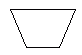Email us to get an instant 20% discount on highly effective K-12 Math & English kwizNET Programs!

#### Online Quiz (WorksheetABCD)

Questions Per Quiz = 2 4 6 8 10

### Grade 5 - Mathematics7.20 Plane Figures Review Test

 Q 1: Find the area of a rectangle 10 cm by 84 cm.840 sq. cm2,352 sq. cm224 sq. cm Q 2: I have an obtuse angle and 3 equal sides. What am I?Equilateral Acute TriangleScalene Obtuse TriangleObtuse Isosceles TriangleEquilateral Obtuse Triangle Q 3: What is the name of this shape?heptagonpentagonhexagonoctagon Q 4: What is the name of this shape?parallelogramsquaretrapizoidrectangle Q 5: A figure that can be folded in half so that the 2 halves match issymmetricala polygonbenchmark Q 6: Obtuse angles are angles that are<90 degrees= 90 degrees>90 degrees Q 7: Examples of quadrilaterals aretrianglelineline segmentsquares and rectangles Q 8: A triangle with 2 sides of equal length is calledscalene triangleequilateral triangleisosceles triangle Question 9: This question is available to subscribers only! Question 10: This question is available to subscribers only!